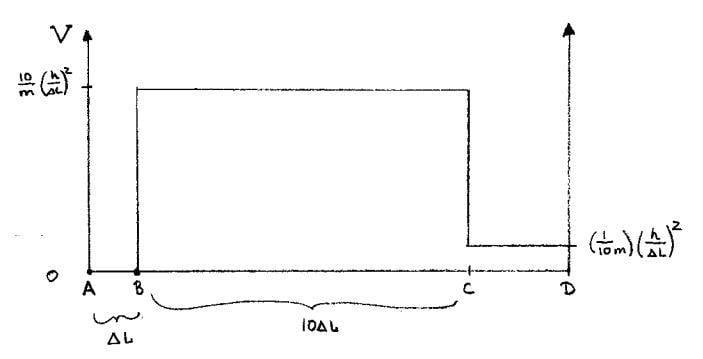# Infinite Square Well, Potential Barrier and Tunneling

• spaghetti3451

## Homework Statement

Consider a one-dimensional, non-relativistic particle of mass ##m## which can move in the three regions defined by points ##A##, ##B##, ##C##, and ##D##. The potential from ##A## to ##B## is zero; the potential from ##B## to ##C## is ##\frac{10}{m}\bigg(\frac{h}{\Delta L}\bigg)^{2}##; and the potential from ##C## to ##D## is ##\frac{1}{10m}\bigg(\frac{h}{\Delta L}\bigg)^{2}##. The distance from ##A## to ##B## is ##\Delta L##; the distance from ##B## to ##C## is ##10 \Delta L##; and the distance from ##C## to ##D## is chosen such that the ground state has the same probability between ##A## and ##B## as between ##C## and ##D##.

(a) For the ground state, the physics of this potential is very close to that of two independent infinite square wells. Why? Exploit this fact to estimate the distance from ##C## to ##D##.

(b) Sketch the ground state wave function, indicating the relative signs in the three regions and the rough relative amplitudes (e.g., which is bigger).

(c) Now consider how to obtain the exact solution. Express the ground state wave function in terms of four normalisation constants, plus the energy and the distance from ##C## to ##D##. Write down equations which determine the six unknowns but do not attempt to solve them. However, you should explicitly compute all integrals and derivatives.## The Attempt at a Solution

(a) For states with higher energies than that for the ground state, the system cannot be well approximated by two independent infinite square wells, because with higher energies the tunneling probability across the potential barrier from ##B## to ##C## is increased. This explains why, for excited states, we cannot approximate the physics of this potential by two independent infinite square wells.

But this does not explain why, for the ground state, the approximation is valid. To demonstrate the validity of this approximation, we need to show that the ground state energy is much much less than the energy of the potential barrier. The ground state energy of the infinite square well with barrier thickness ##\Delta L## is ##\frac{h^{2}}{8m(\Delta L)^{2}}##, whereas the height of the potential barrier is ##\frac{10h^{2}}{m(\Delta L)^{2}}##. So, for the ground state, the physics of this potential is very close to that of two independent infinite square wells.

Am I correct so far?

Last edited: